#### Simple projection sensors

Several common sensor models can be defined by observing particular coordinates ofwhile leaving others invisible. This is the continuous version of the selective sensor from Example 11.4. Imagine, for example, a mobile robot that rolls in a 2D world,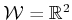, and is capable of rotation. The state space (or configuration space) is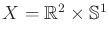. For visualization purposes, it may be helpful to imagine that the robot is very tiny, so that it can be interpreted as a point, to avoid the complicated configuration space constructions of Section 4.3.11.7 Let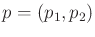denote the coordinates of the point, and let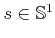denote its orientation. Thus, a state in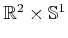is specified as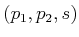(rather than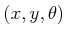, which may cause confusion with important spaces such as,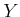, and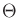).

Suppose that the robot can estimate its position but does not know its orientation. This leads to a position sensor defined as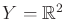, with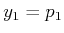and(also denoted as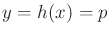). The third state variable,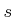, of the state remains unknown. Of course, any of the previously considered nature sensing action models can be added. For example, nature might cause errors that are modeled with Gaussian probability densities.

A compass or orientation sensor can likewise be made by observing only the final state variable,. In this case,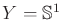and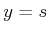. Nature sensing actions can be included. For example, the sensed orientation may be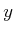, but it is only known that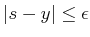for some constant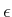, which is the maximum sensor error. A Gaussian model cannot exactly be applied because its domain is unbounded and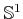is bounded. This can be fixed by truncating the Gaussian or by using a more appropriate distribution.

The position and orientation sensors generalize nicely to a 3D world,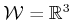. Recall from Section 4.2 that in this case the state space is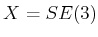, which can be represented as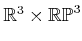. A position sensor measures the first three coordinates, whereas an orientation sensor measures the last three coordinates. A physical sensor that measures orientation in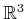is often called a gyroscope. These are usually based on the principle of precession, which means that they contain a spinning disc that is reluctant to change its orientation due to angular momentum. For the case of a linkage of bodies that are connected by revolute joints, a point in the state space gives the angles between each pair of attached links. A joint encoder is a sensor that yields one of these angles.

Dynamics of mechanical systems will not be considered until Part IV; however, it is worth pointing out several sensors. In these problems, the state space will be expanded to include velocity parameters and possibly even acceleration parameters. In this case, a speedometer can sense a velocity vector or a scalar speed. Sensors even exist to measure angular velocity, which indicates the speed with which rotation occurs. Finally, an accelerometer can be used to sense acceleration parameters. With any of these models, nature sensing actions can be used to account for measurement errors.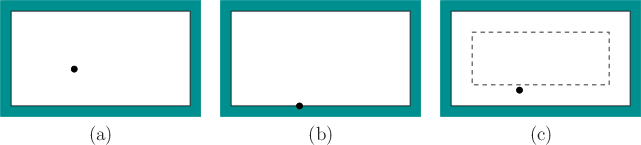Steven M LaValle 2020-08-14